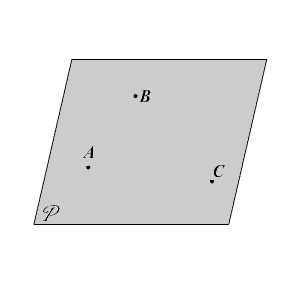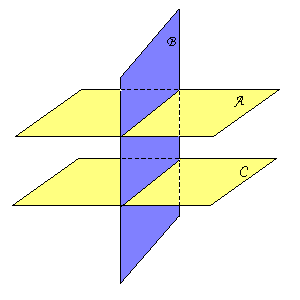# Planes

A plane is a flat surface that extends infinitely in all directions. Given any three non-collinear points, there is exactly one plane through them.

A plane can be named by a capital letter, often written in script, or by the letters naming three non-collinear points in the plane.

For example, the plane in the diagram below could be named either plane $ABC$ or plane $P$ .Two planes can be parallel (planes $A$ and $C$ in the figure below), or they can intersect in a line (planes $A$ and $B$ .)